• Slides: 27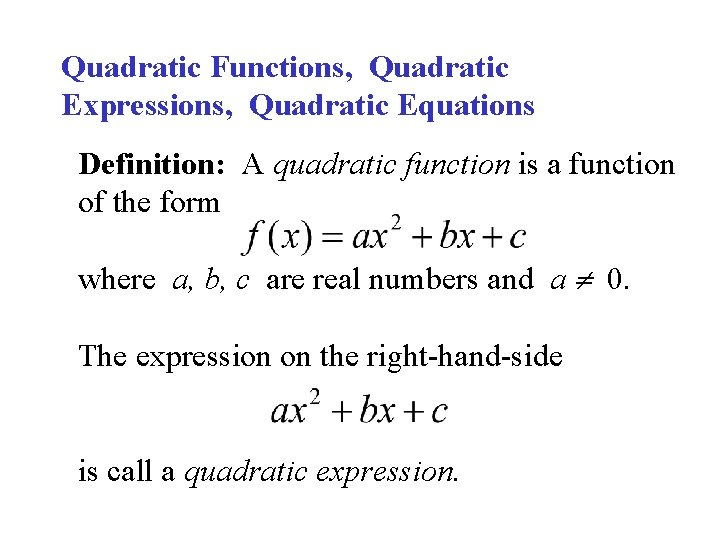Quadratic Functions, Quadratic Expressions, Quadratic Equations Definition: A quadratic function is a function of the form where a, b, c are real numbers and a 0. The expression on the right-hand-side is call a quadratic expression.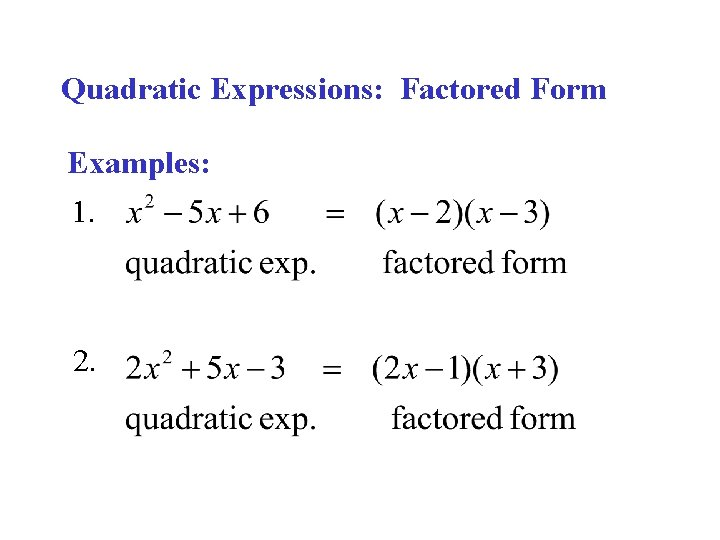Quadratic Expressions: Factored Form Examples: 1. 2.Factoring quadratic expressions: Given integers. Case 1: a = 1; Since where a, b, c are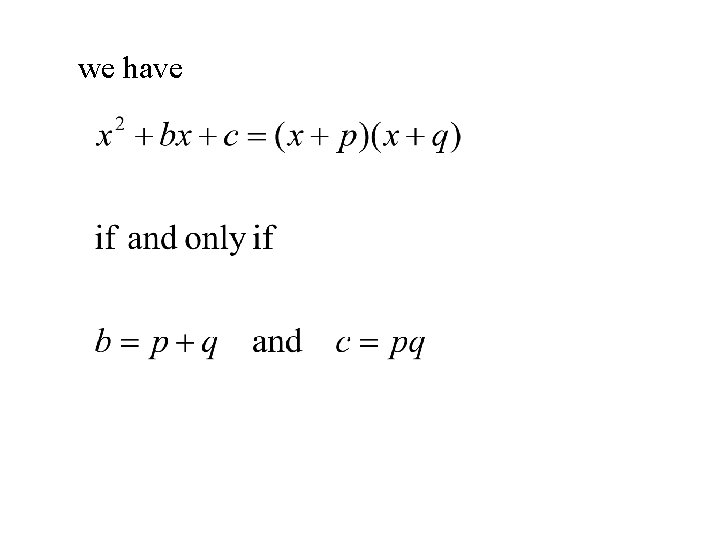we haveExamples:Case 2: integers and a 1. Since where a, b, c are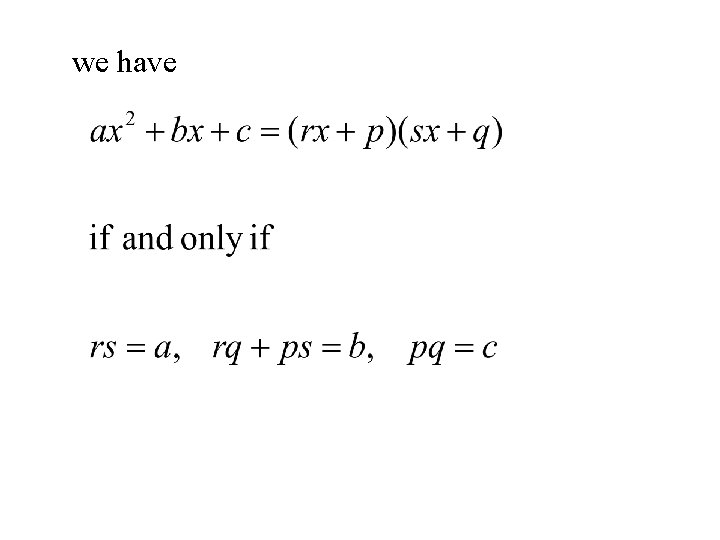we have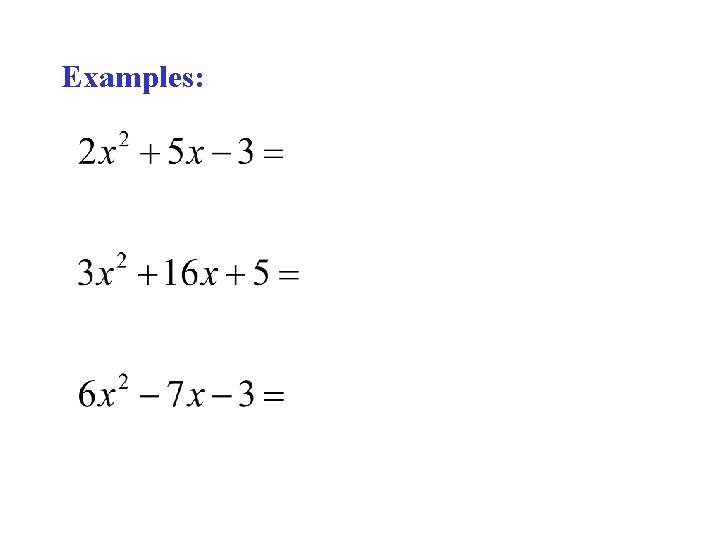Examples:Quadratic Equations: A quadratic equation is an equation of the form: Problem: Find the real numbers x, if any, that satisfy the equation. The numbers that satisfy the equation are called solutions or roots.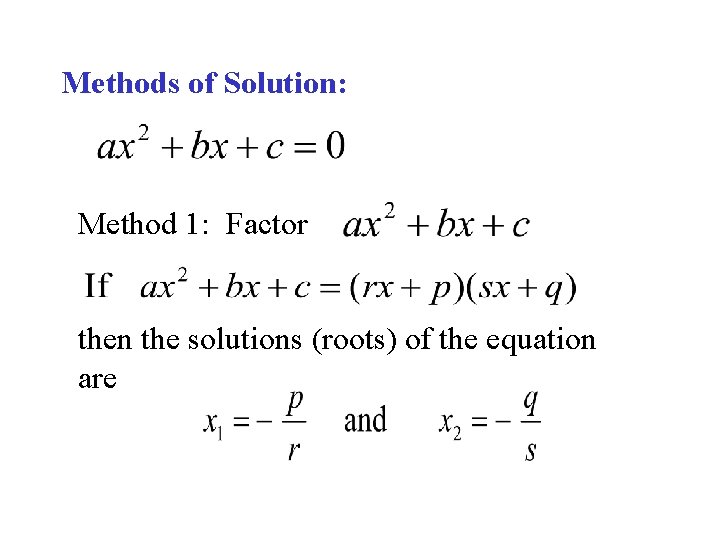Methods of Solution: Method 1: Factor then the solutions (roots) of the equation areExamples: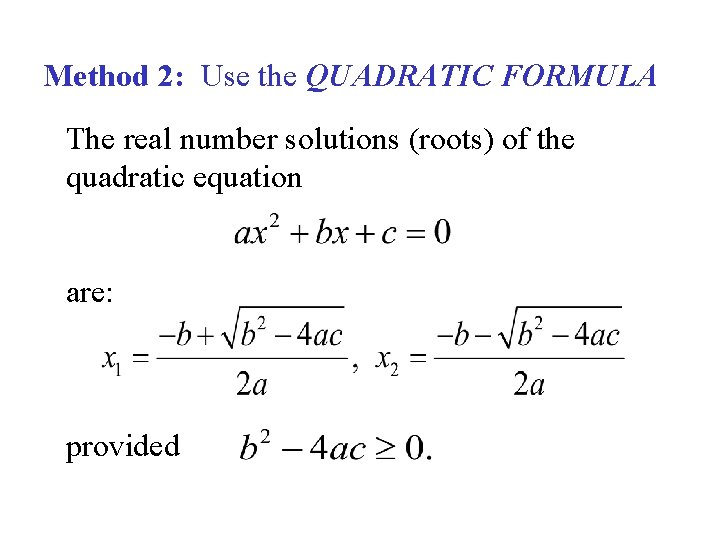Method 2: Use the QUADRATIC FORMULA The real number solutions (roots) of the quadratic equation are: providedThe quadratic formula is often written as The number discriminant. is called theThe Discriminant: Given the quadratic equation If:(1) ; the roots are: (2) the roots are: (3) no real roots.Examples: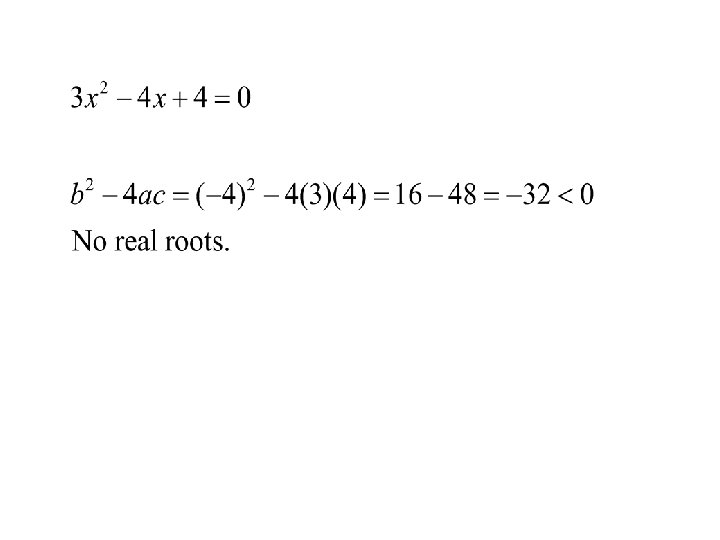Quadratic Functions: The graph of is a parabola. The graph looks like if a > 0 if a < 0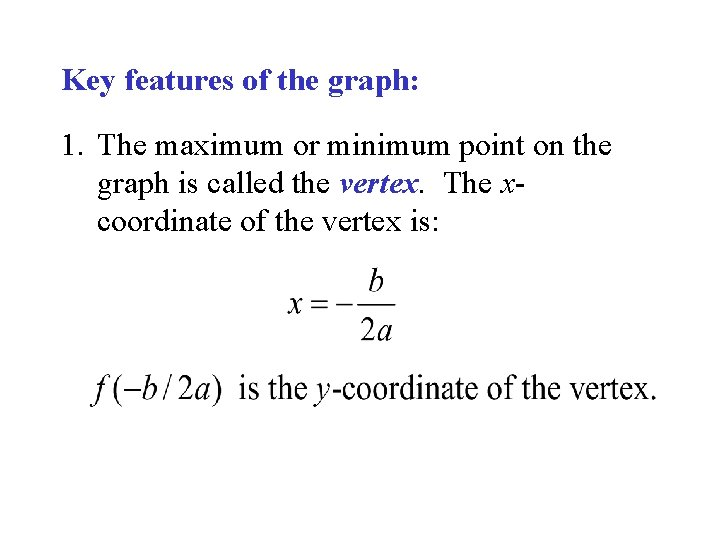Key features of the graph: 1. The maximum or minimum point on the graph is called the vertex. The xcoordinate of the vertex is: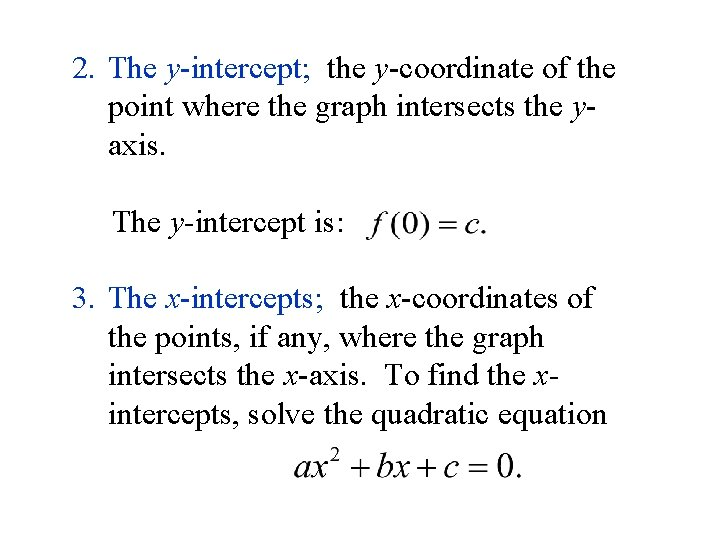2. The y-intercept; the y-coordinate of the point where the graph intersects the yaxis. The y-intercept is: 3. The x-intercepts; the x-coordinates of the points, if any, where the graph intersects the x-axis. To find the xintercepts, solve the quadratic equation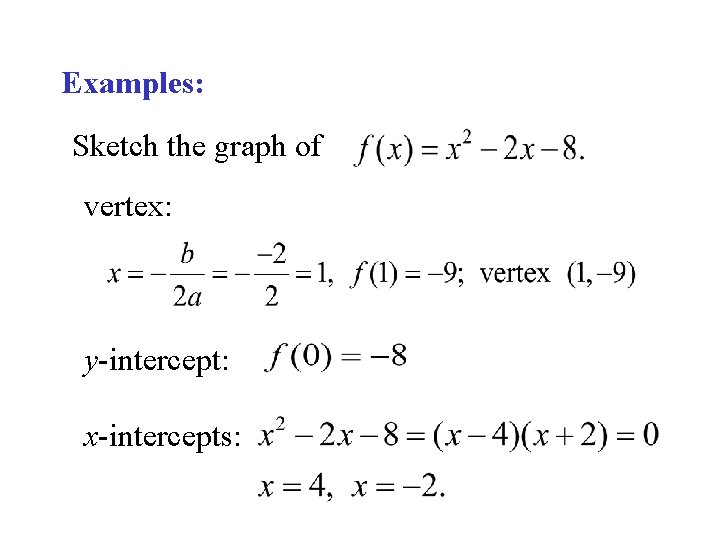Examples: Sketch the graph of vertex: y-intercept: x-intercepts: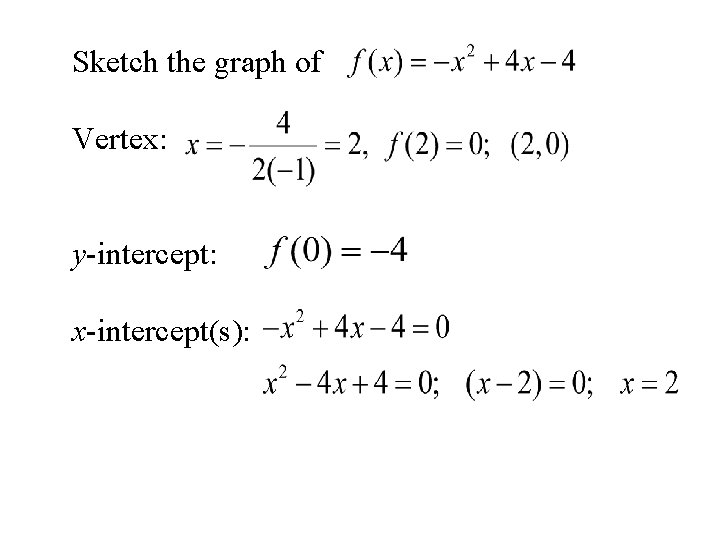Sketch the graph of Vertex: y-intercept: x-intercept(s):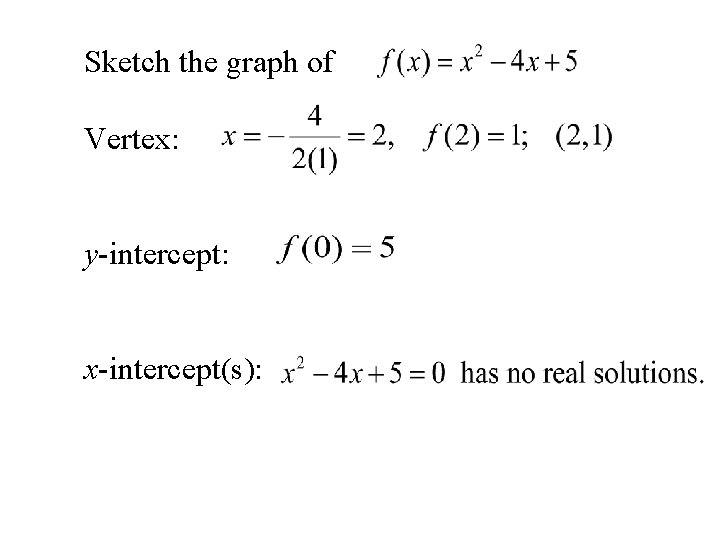Sketch the graph of Vertex: y-intercept: x-intercept(s):